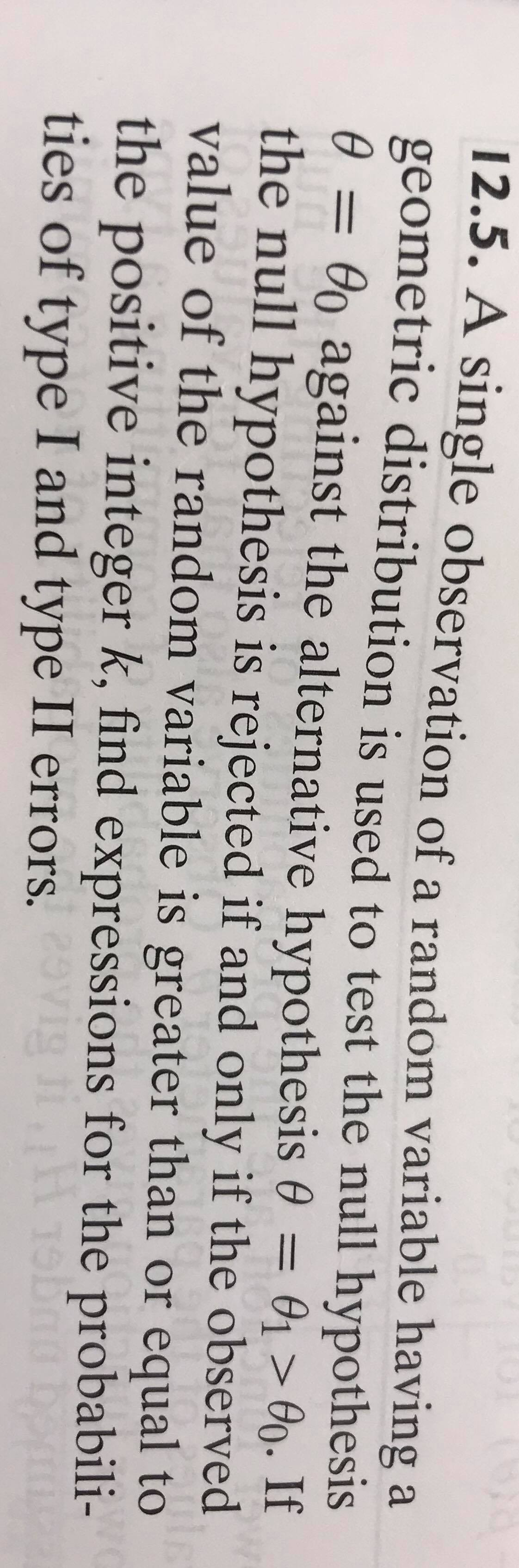# 12.5. A single observation of a random variable having ageometric distribution is used to test the null hypothesis0 = 0o against the alternative hypothesis 0 = 01 > 09. Ifthe null hypothesis is rejected if and only if the observedvalue of the random variable is greater than or equal tothe positive integer k, find expressions for the probabili-ties of type I and type II errors.%3Dvig

Question
30 viewshelp_outlineImage Transcriptionclose12.5. A single observation of a random variable having a geometric distribution is used to test the null hypothesis 0 = 0o against the alternative hypothesis 0 = 01 > 09. If the null hypothesis is rejected if and only if the observed value of the random variable is greater than or equal to the positive integer k, find expressions for the probabili- ties of type I and type II errors. %3D vig fullscreen
check_circle

Step 1

Introduction:

The probability mass function (pmf) and cumulative distribution function (cdf) of a random variable, X, having a geometric distribution with parameter, θ are as follows:

PMF: f (x) = (1 – θ) x–1 θ; if x = 1, 2, …; f (x) is 0 , otherwise.

CDF: F (x) = 1 – (1 – θ...

### Want to see the full answer?

See Solution

#### Want to see this answer and more?

Solutions are written by subject experts who are available 24/7. Questions are typically answered within 1 hour.*

See Solution
*Response times may vary by subject and question.
Tagged in

### Random Variables